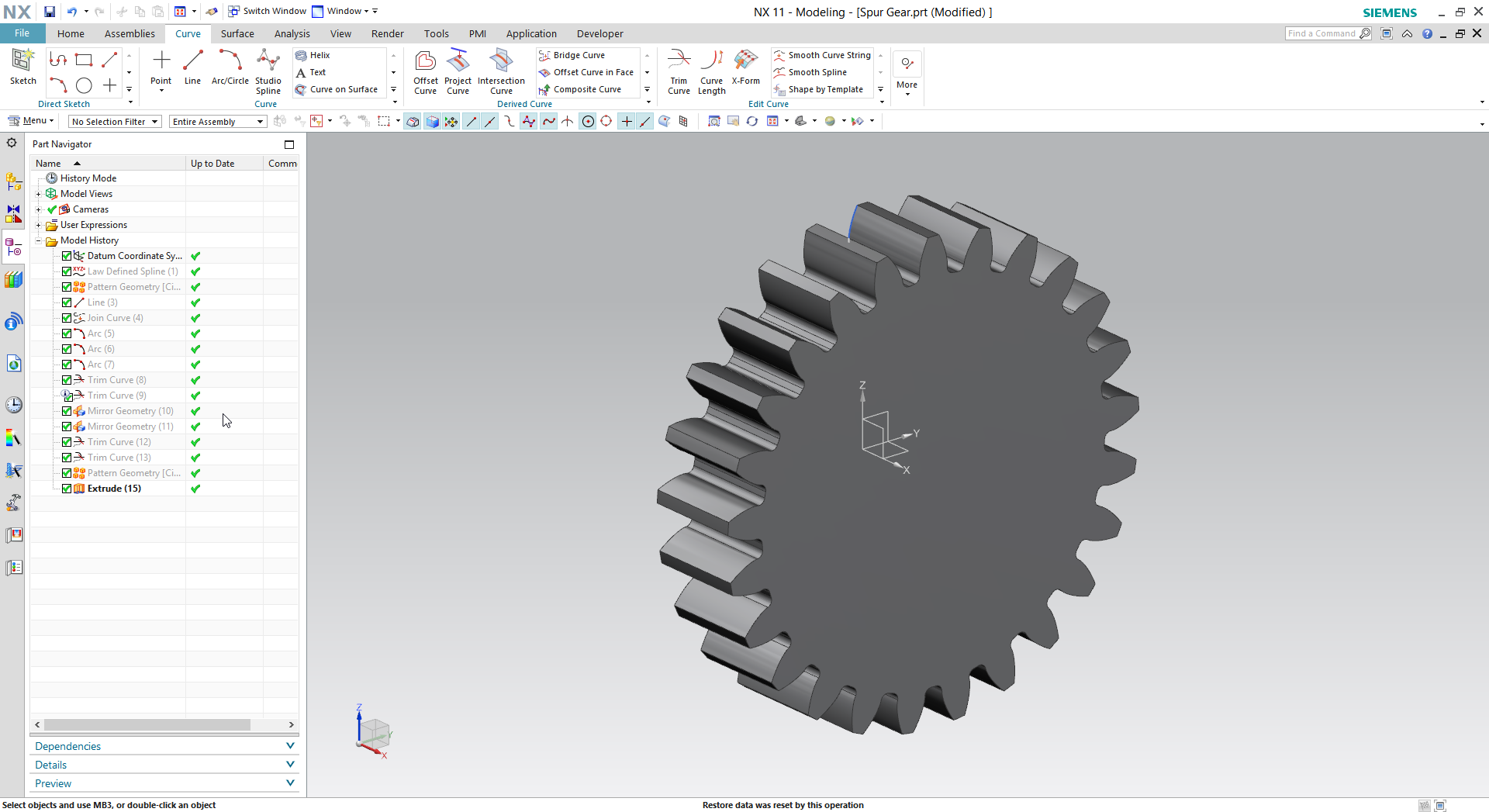# How to create spur gear in Siemens NX?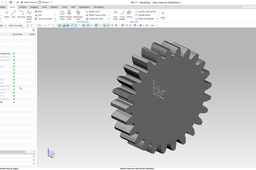This tutorial presents the modeling of an external spur gear in Siemens NX.
The detailed modeling process is demonstrated on this video:

https://youtu.be/pVPjDyOn6R4

UPDATE: At ~ 2:40 leave the Limit options at default (At Point). See the errata video for the reason:
https://youtu.be/PUpdlyQcaOs

1. ### Step 1:

Copy these parameters into a text file and save it. Rename the file extension to .exp.

[degrees]alpha=20 //Reference Pressure Angle
c=sqrt(1/(cos(alpha))^2-1)/pi() //Parameter of Involute Curve
[mm]m=3.5 //Module
[degrees]phi=arctan(yc/zc)+90/z //Rotation angle
t=0 //NX Parameter
[mm]xt=0 //x Coordinates of Involute
yc=rb*(sin(deg(c*pi()))-cos(deg(c*pi()))*c*pi())
yt=rb*(sin(deg(t*pi()))-cos(deg(t*pi()))*t*pi()) //y Coordinates of Involute
(Integer) z=25 //Number of Teeth
zc=rb*(cos(deg(c*pi()))+sin(deg(c*pi()))*c*pi())
zt=rb*(cos(deg(t*pi()))+sin(deg(t*pi()))*t*pi()) //z Coordinates of Involute

2. ### Step 2:

Launch NX, create a new model file, push the CTRL+E keys and imports the expressions.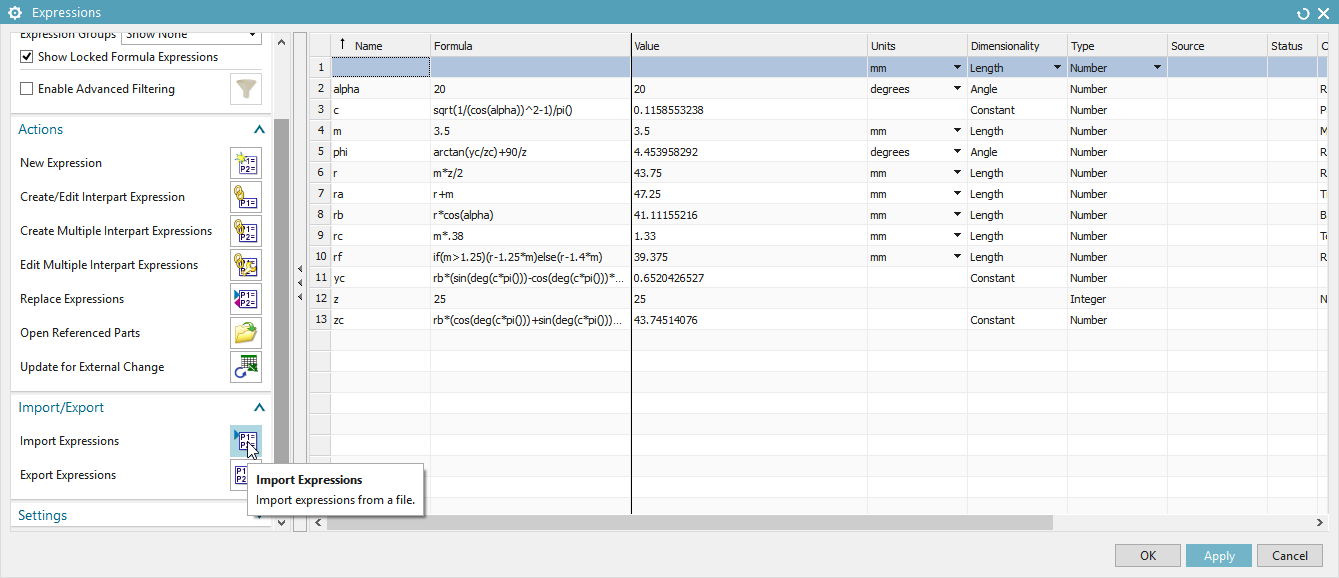3. ### Step 3:

Create the involute curve by Law Curve command.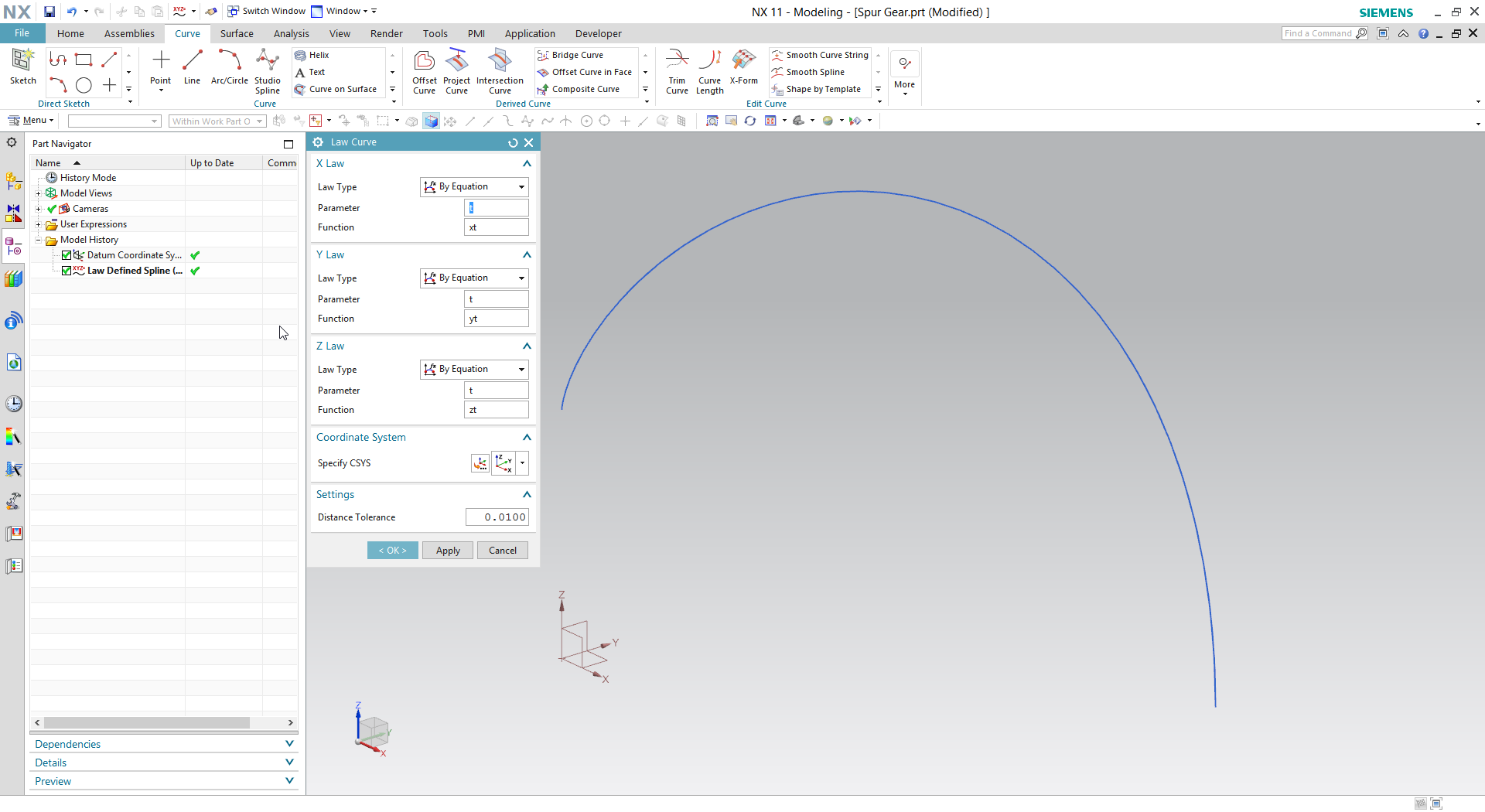4. ### Step 4:

Create a circular pattern on the involute curve.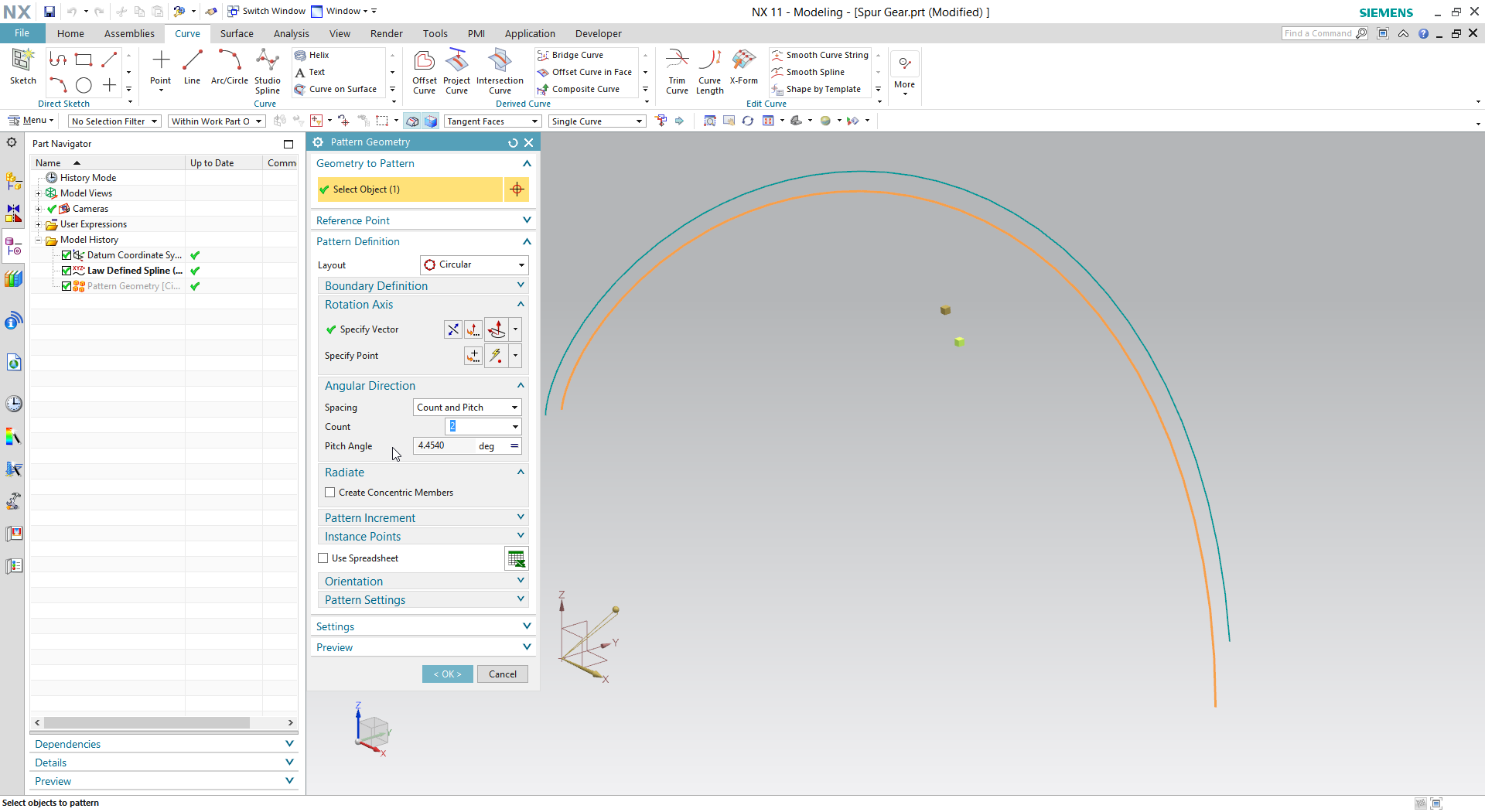5. ### Step 5:

Draw a line which starts from the end point of involute and tangents the curve. Set its limit by equation.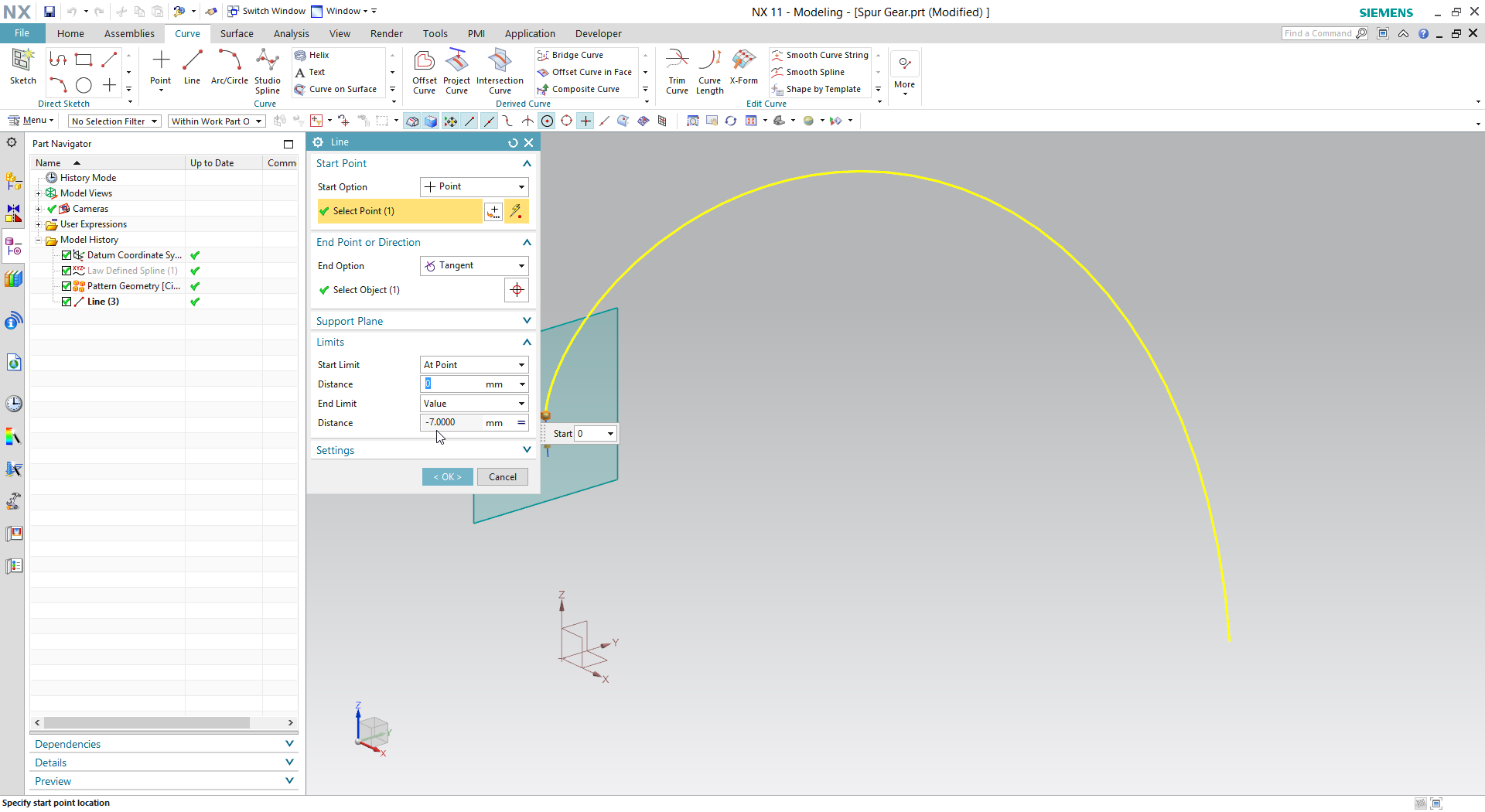6. ### Step 6:

Launch the Join Curve command and join the line and the involute curve.

7. ### Step 7:

Draw the tip and root circles by full circle. Draw a tangent circle for the tooth blend.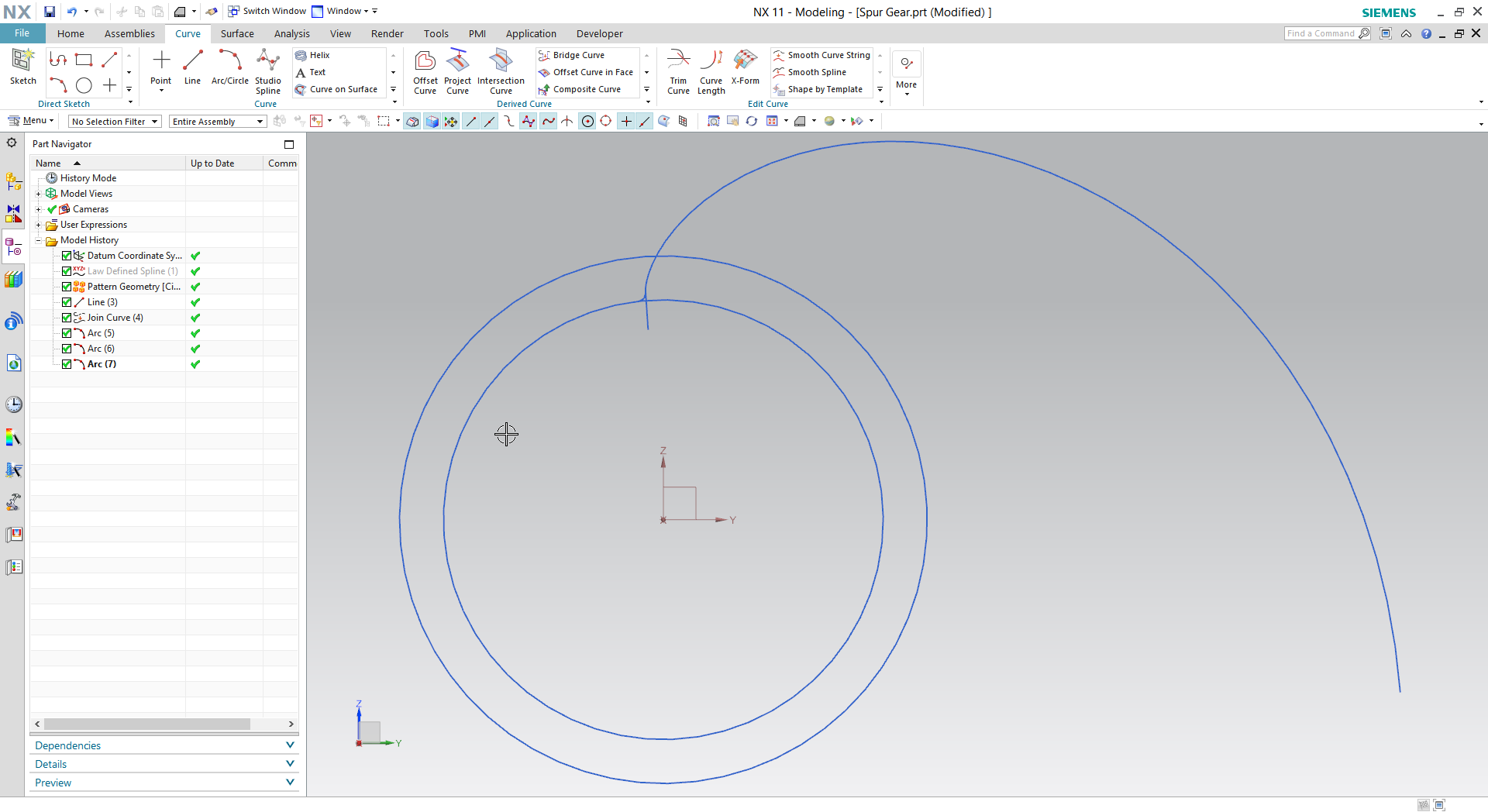8. ### Step 8:

Trim the unnecessary parts of the curves.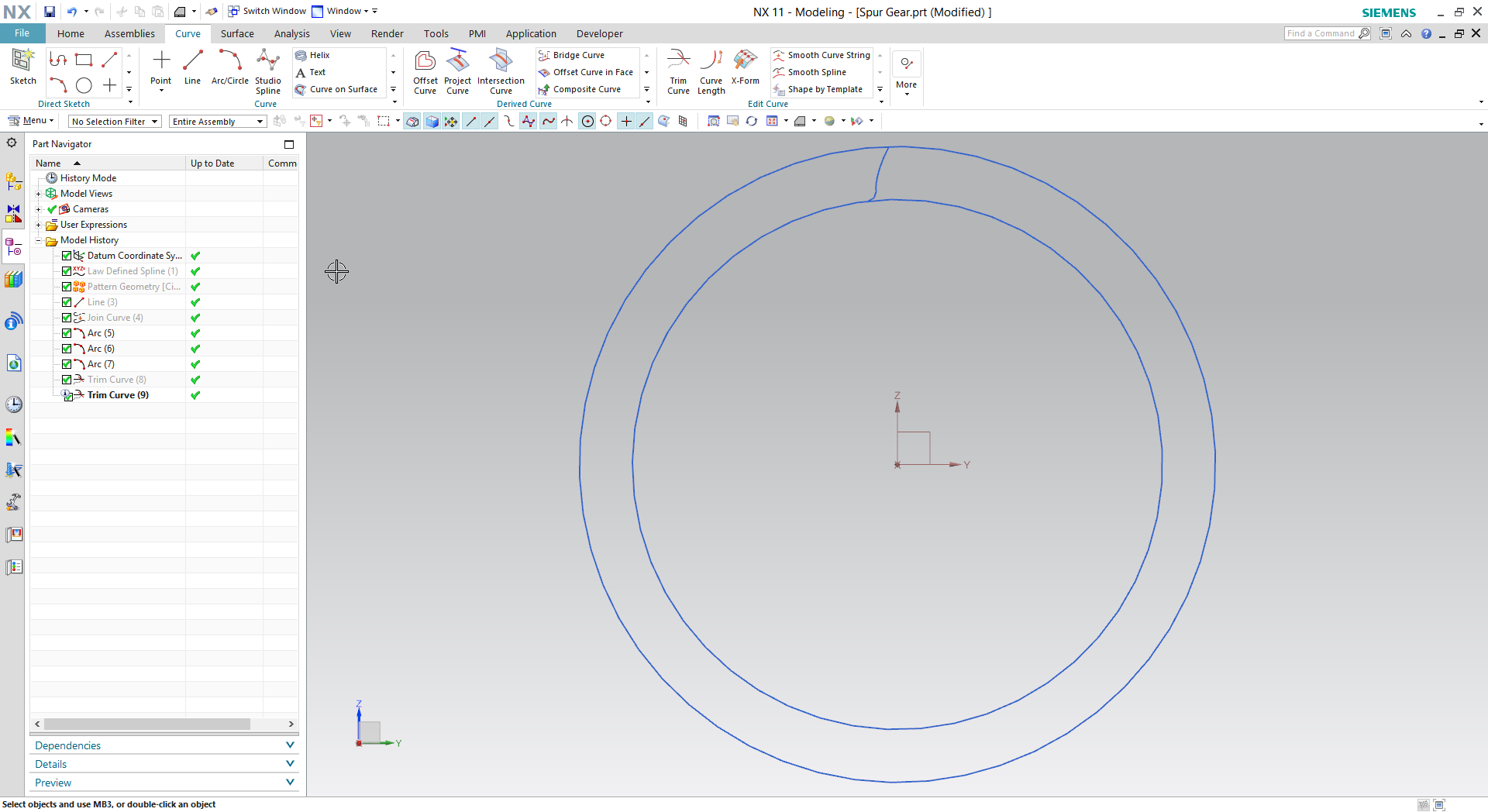9. ### Step 9:

Mirror the involute curve and the tangent circle.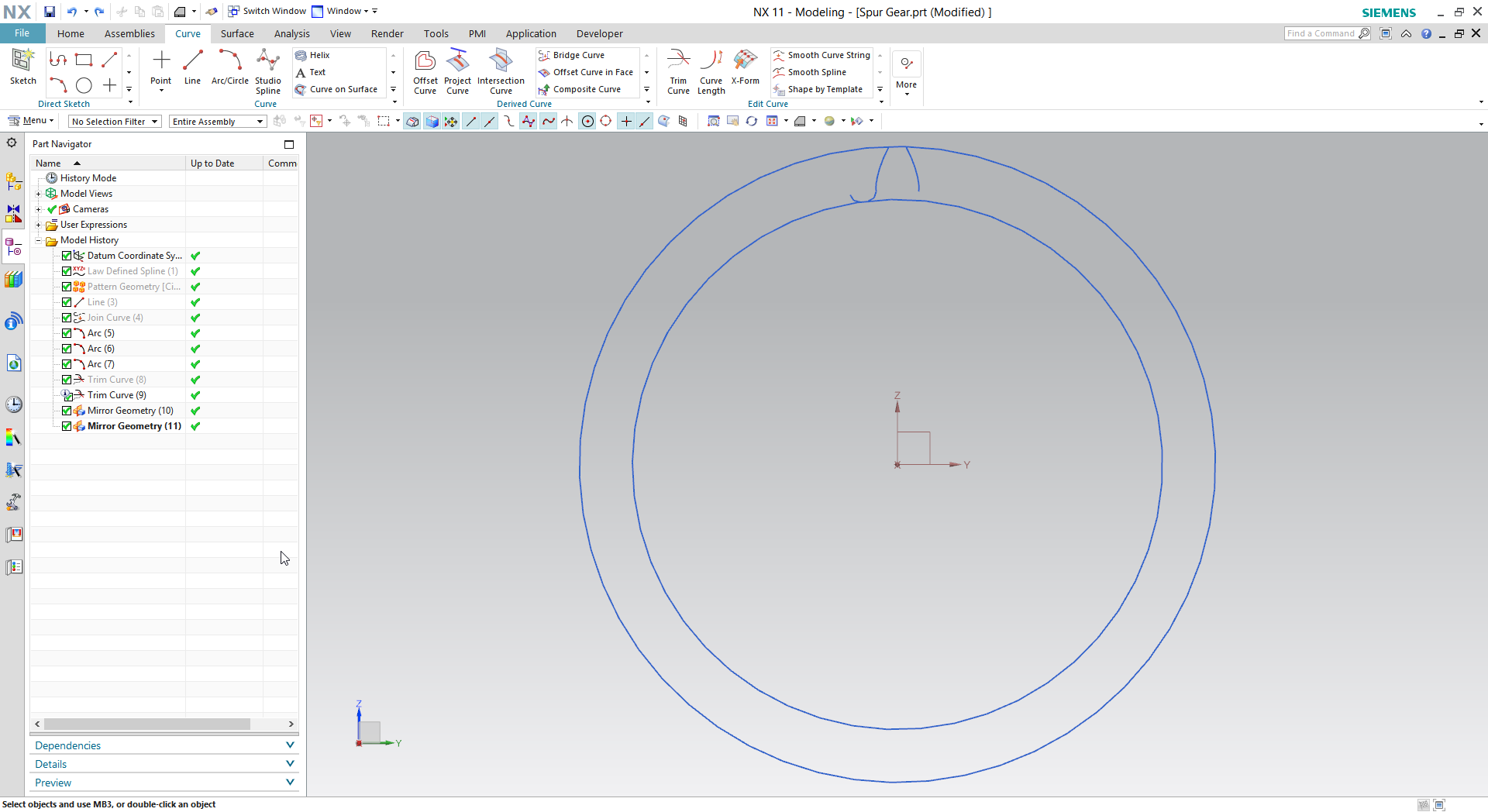10. ### Step 10:

Trim the tip and root circles.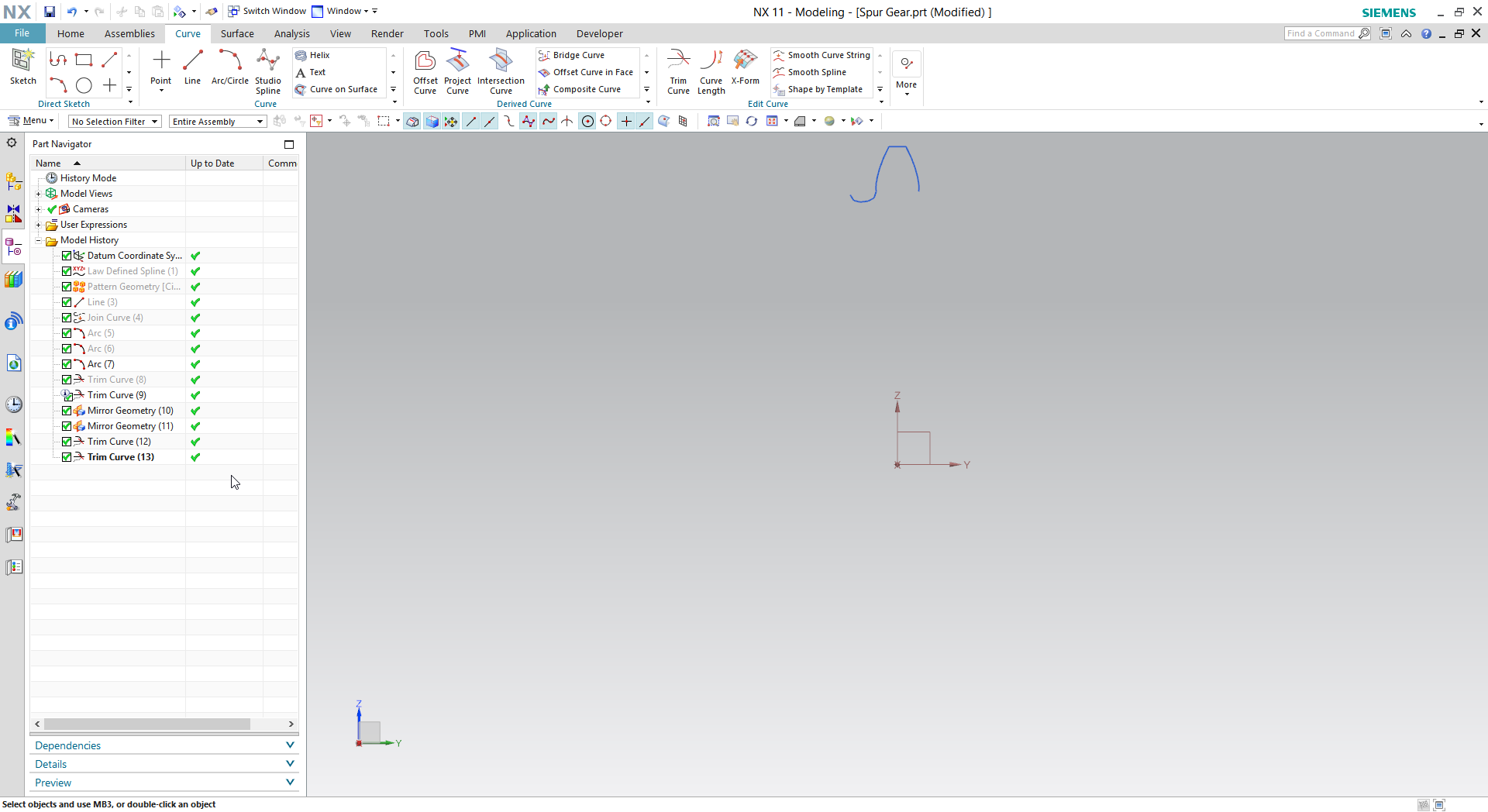11. ### Step 11:

Create a circular pattern, set the parameters.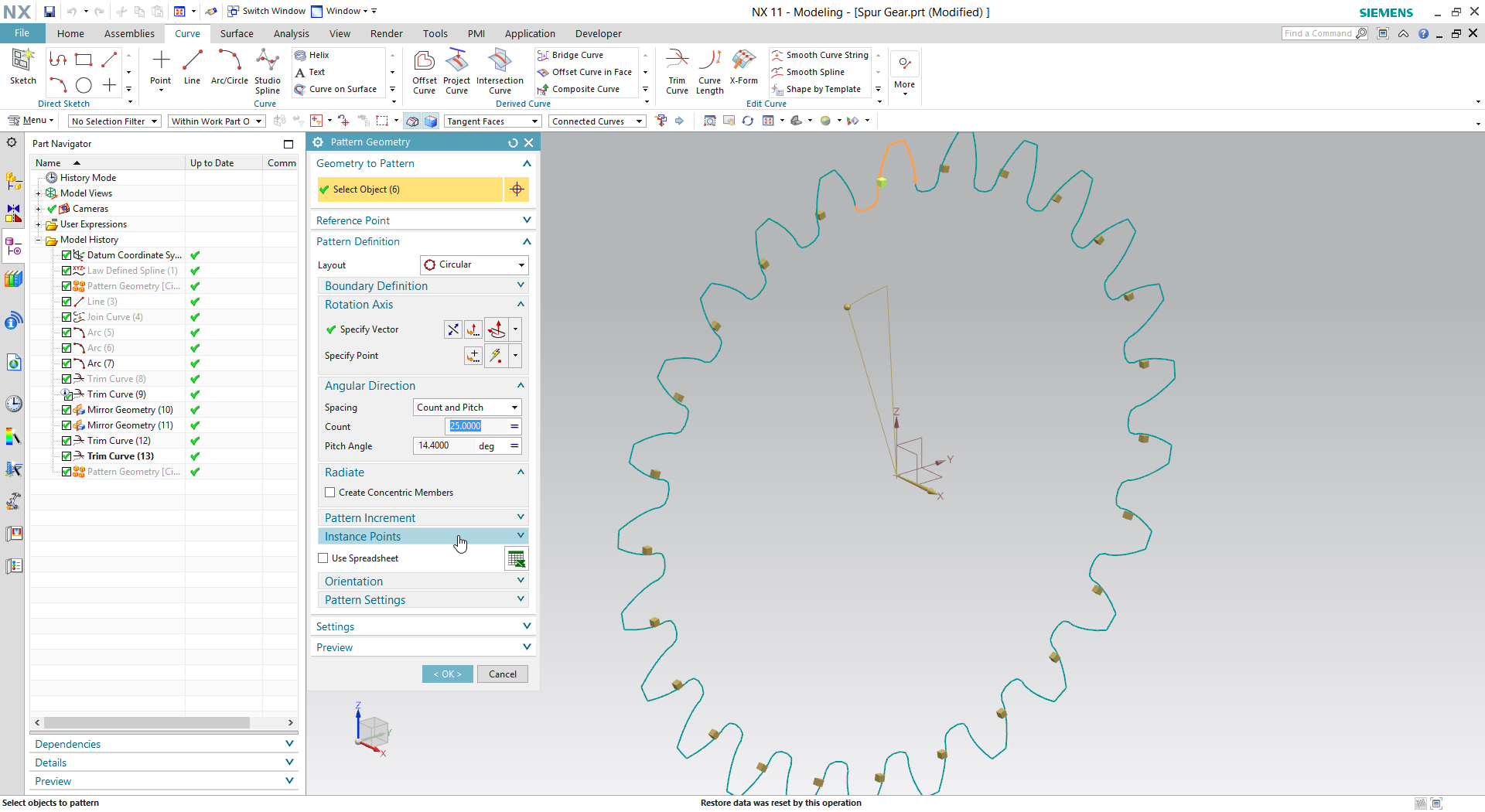12. ### Step 12:

Extrude the curves to get the spur gear body.